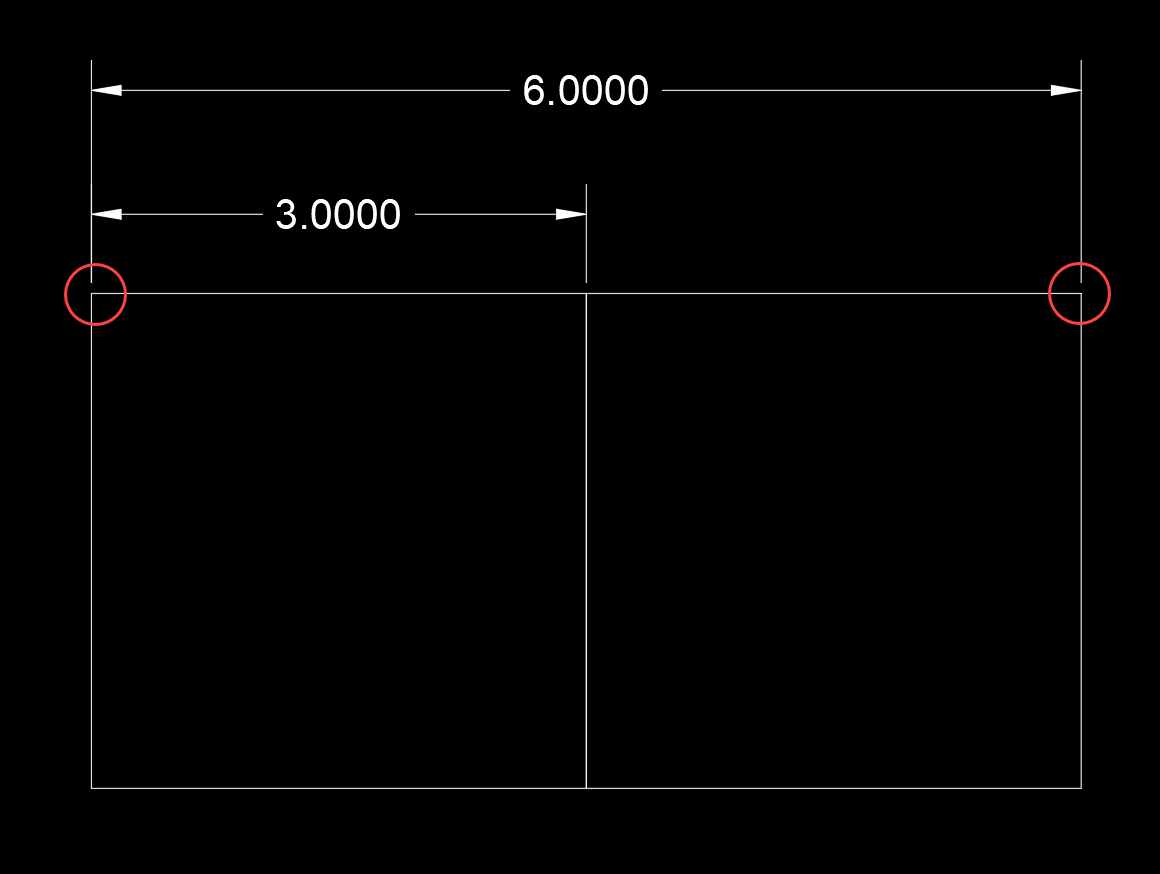# Measure, Distance, and Area commands not giving correct values

Issue:
You use the Measure, Distance, or Area command and get a value that is not correct

Causes:

This problem is usually because you have items at different Z elevations, and are using Object Snaps (OSNAPS) to select start and end points with different Z Values

Example
A linear dimension between the two points (red circle) shows 6.000 unitsHowever, when I use the distance command between them, I get this:
Specify first point:
Specify second point or [Multiple points]:
Distance = 6.7082,  Angle in XY Plane = 0,  Angle from XY Plane = 27
Delta X = 6.0000,  Delta Y = 0.0000,   Delta Z = 3.0000

This is because the right square is at a Z elevation of 3, and the left square is at a Z elevation of 0

Solution:
If you are using OSNAPS, then your best bet is to turn off the OSNAPZ:
OSNAPZ:
Controls whether object snaps are automatically projected onto a plane parallel to the XY plane of the current UCS at the current elevation.

Value    Description
0        Osnap uses the Z -value of the specified point
1        Osnap substitutes the Z -value of the specified point with the elevation (ELEV) set for the current UCS

This is NOT saved, so each time you open a new drawing or close and re-open AutoCAD, you will need to change this value back

###### Previous ArticleInventor Part Density/Mass Shows a “0.000” Value After Upgrade

Solution for Inventor parts that show a "0" value Density/Mass iProperty value.

###### Next ArticleAutodesk Point Layout Basics

Get the basics of Point Layout. Stay tuned for future articles that will address more advanced features.